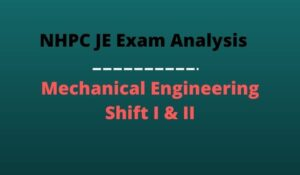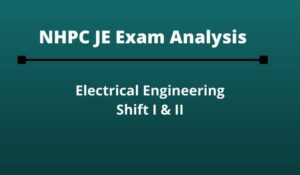Engineering Jobs   »   Civil Engineering quizs   »   NHPC-JE

# NHPC-JE’21 CE: Daily Practices Quiz. 30-Sep-2021

Know your strengths and practice your concepts with this quiz on NHPC JE Recruitment 2021. This quiz for NHPC JE Recruitment 2021 is designed specially according to NHPC Syllabus 2021.

Quiz: Civil Engineering
Exam: NHPC-JE
Topic: Miscellaneous

Each question carries 1 mark
Negative marking: 1/4 mark
Time: 8 Minutes

Q1. If the R.L. of canal bed level and high flood level of drainage are 212.0 m and 210 m respectively, then the cross-drainage work will be
(a) Aqueduct
(b) Syphon
(c) Syphon aqueduct
(d) Super passage

Q2. Minimum pitch of rivets shall not be less than
(a) 1.5 d
(b) 2.0 d
(c) 2.5 d
(d) 3.0 d

Q3. A simply supported beam is considered as a deep beam is the ratio of effective span to overall depth is less than
(a) 1
(b) 2
(c) 3
(d) 4

Q4. The maximum safe permissible limit of sulphates in domestic water supply is
(a) 200 mg/l
(b) 600 mg/l
(c) 100 mg/l
(d) 500 mg/l

Q5. Calculate the length (m.) of the longer chord of 260 m radius curve having deflection angle of 90 degree.
(a) 353.6 m
(b) 392.7 m
(c) 367.7 m
(d) 500 m

Q6. A soil has bulk density of 22 kN/m³ and water content 10%. The dry density is
(a) 20 kN/m³
(b) 15 kN/m³
(c) 18 kN/m³
(d) 19 kN/m³

Solutions

S1. Ans.(a)
Sol. Given, canal bed level = 212.0 m.
High flood level of drainage = 210.0 m.
Here
Bed level of canal is quite higher than high flood level of drainage, hence the cross-drainage work will be aqueduct.

S2. Ans.(c)
Sol. → minimum pitch of rivet = 2.5d
→ maximum pitch for compression = (12t,200)_minimum
→ maximum pitch for tension = (16t,200)_minimum

S3. Ans.(b)
Sol. According to clause 29.1 or IS 456 : 2000
A beam shall be deemed to be deep beam when the ratio of effective span to overall depth l/D is less than:
1. 2.0 for a simply supported beam; and
2. 2.5 for a continuous beam.

S4. Ans.(a)
Sol. The maximum safe-limits in domestic water supply are:
Chloride ≤ 250 mg/l
Nitrates ≤ 45 mg/l
Sulphates ≤ 200 mg/l
Fluorides ≤ 1.5 mg/l

S5. Ans.(c)
Sol. Given, Radius of curve (R) = 260 m.
Deflection angle (∆) = 90°
Length of Long chord is given by–
T₁DT₂ = 2R sin ∆/2
= 2 × 260 × sin 90/2
▭(T_1 DT_2=367.7 m)

S6. Ans.(a)
Sol. Given,
γ_b=22 kN\/m^3
w=10%=0.1
γ_d= ?
γ_d=γ_b/(1+w)
=22/(1+0.1)
=22/1.1
▭(γ_d=20 kN\/m^3 )

Sharing is caring!

### TOPICS:

•NHPC Junior Engineer Syllabus 2023, Chec...
•NHPC JE Exam Pattern 2023, Latest Markin...
•NHPC JE Syllabus 2023, Download Latest S...
•NHPC Result Through GATE 2021, Download ...
•NHPC JE Mechanical Exam Analysis 2022 Di...
•NHPC JE Exam Analysis 2022 Electrical En...